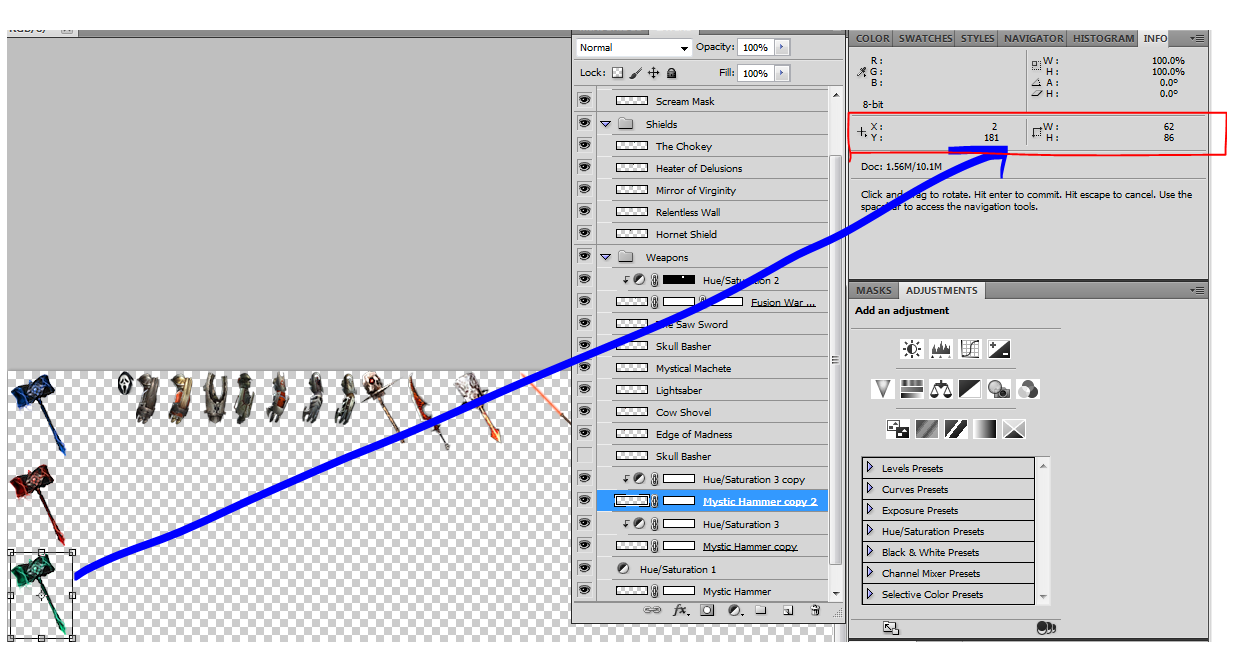# How to copy data to the clipboard from the info panel?

For example:I am wanting to copy some data from the info panel, preferably `x, y, width and height` to my clipboard. Is this possible within Photoshop? The reason I ask is because I have hundreds of items that I need to grab coordinates from.

No problem we can script this in a minute (10 to be exact, I’m a bit rusty). Put this script in a .jsx  file and run it using extend script toolkit:

``````#target photoshop

main();

function main() {
var layers = app.activeDocument.layers;
var file = File.saveDialog('save layer info', 'layerinfo:*.txt');
file.open('w');
handleOnelayer(layers, file, "");
file.close();
}

function handleOnelayer(layers, file, pf){
var numLayers = layers.length;
for (var j = 0; j < numLayers; j++) {
var currentLayer = layers[j];

if (currentLayer.typename == 'ArtLayer'){
var bounds = currentLayer.bounds;
var name = currentLayer.name;
var width = bounds - bounds;
var height = bounds - bounds;

file.write(
pf + name+":\n" +
pf +       "    X = " + bounds + "\n"+
pf +       "    Y = " + bounds + "\n"+
pf +       "    W = " + width + "\n"+
pf +       "    H = " + height + "\n\n"
);
} else if (currentLayer.typename == 'LayerSet'){
var name = currentLayer.name;
file.write(
pf +"--  " + name+"  --\n"
);
handleOnelayer(currentLayer.layers,
file,
pf + "    ");
}
}
}
``````

It will ask for a file name where to write the X, Y, W and H data. Example output:

``````--  Group 1  --
Layer 2:
X = 245 px
Y = 103 px
W = 786 px
H = 366 px

Layer 1:
X = 1360 px
Y = 382 px
W = 47 px
H = 139 px

Background:
X = 0 px
Y = 0 px
W = 1913 px
H = 1200 px
``````